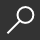## Mathco College Algebra : The Step-by-Step Study Guide by L. Muriel Locke

Overview -
Need help with your algebra course? Then Mathco College Algebra was written just for you. This study guide can help you to lock-in all of the fundamental concepts of College Algebra. The study guide gives you easy-to-learn Math notes and definitions, rewritten to help you understand the concepts. Each chapter shows you completely worked, step-by-step examples for each lesson presented. This study guide also provides you with study tips and helpful hints for working with factoring of polynomials, algebraic fractions, radicals, logarithms, and more. The topics include linear equations and word problems, functions and graphs, equations and word problems involving polynomials, algebraic fractions, radicals, logarithms, and exponential expressions. The study guide also covers linear and non-linear inequalities, linear systems, factorials, counting theory, and the binomial theorem. Mathco College Algebra is especially helpful for students in the following courses: College Algebra, Precalculus, Math Analysis, Math Concepts, Algebra II, Developmental Math II, Math Essentials (Units 6-9). It may be also be used as a reference guide for Applied Calculus, and Calculus with Analytic Geometry. Use Mathco College Algebra as your personal Math partner to achieve greater success in your Math course.

local_shippingFor DeliveryIn Stock.
This item is Non-Returnable.
FREE Shipping for Club Members helpNew & Used Marketplace 3 copies from \$19.39

## More About Mathco College Algebra by L. Muriel Locke

### Overview

Need help with your algebra course? Then Mathco College Algebra was written just for you. This study guide can help you to lock-in all of the fundamental concepts of College Algebra. The study guide gives you easy-to-learn Math notes and definitions, rewritten to help you understand the concepts. Each chapter shows you completely worked, step-by-step examples for each lesson presented. This study guide also provides you with study tips and helpful hints for working with factoring of polynomials, algebraic fractions, radicals, logarithms, and more. The topics include linear equations and word problems, functions and graphs, equations and word problems involving polynomials, algebraic fractions, radicals, logarithms, and exponential expressions. The study guide also covers linear and non-linear inequalities, linear systems, factorials, counting theory, and the binomial theorem. Mathco College Algebra is especially helpful for students in the following courses: College Algebra, Precalculus, Math Analysis, Math Concepts, Algebra II, Developmental Math II, Math Essentials (Units 6-9). It may be also be used as a reference guide for Applied Calculus, and Calculus with Analytic Geometry. Use Mathco College Algebra as your personal Math partner to achieve greater success in your Math course.

This item is Non-Returnable.

### Details

• ISBN-13: 9781717108074
• ISBN-10: 1717108075
• Publisher: Createspace Independent Publishing Platform
• Publish Date: May 2018
• Page Count: 220
• Dimensions: 11 x 8.5 x 0.46 inches
• Shipping Weight: 1.15 poundsRelated Categories